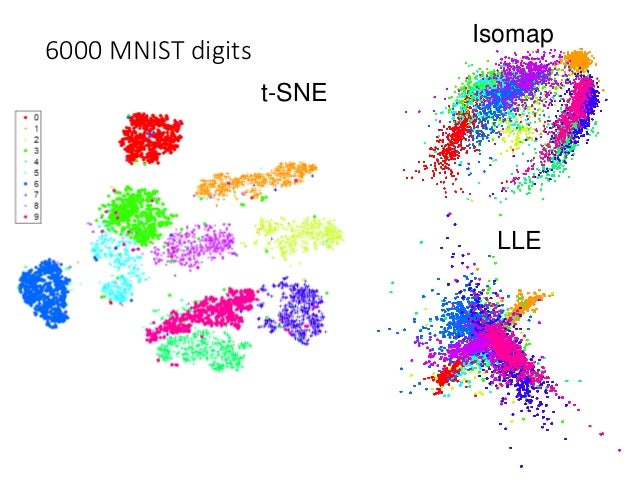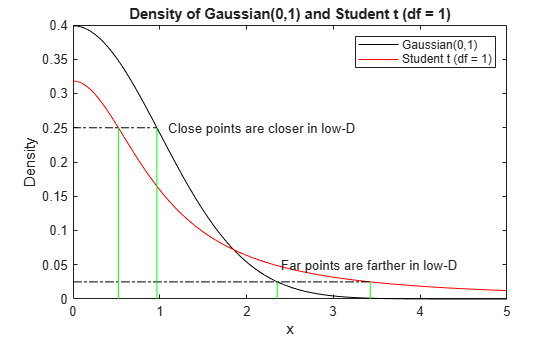3 Matching Annotations
1. Feb 2021
2. constantamateur.github.io constantamateur.github.io

#### URL

3. Nov 2018
4. iphysresearch.github.io iphysresearch.github.io
1. UMAP: Uniform Manifold Approximation and Projection for Dimension Reduction

此文提供了一个和 t-sne 非常类似的降维可视化算法。效果相当不错！也开源了算法代码。

按照作者的说法，UMAP 比 T-SNE 算法更好的优点有二：更快！更准！

#### URL

5. Oct 2018
6. 192.168.199.102:5000 192.168.199.102:5000
1. # T-distribution Stochastic Neighbor Embedding(t-SNE)

之前介绍的所有方法都存在相同的弊病：

similar data are close, but different data may collapse，亦即，相似（label）的点靠的确实很近，但不相似(label)的点也有可能靠的很近。## t-SNE 的原理

$$x \rightarrow z$$

t-SNE 一样是降维，从 x 向量降维到 z. 但 t-SNE 有一步很独特的标准化步骤：

一， t-SNE 第一步：similarity normalization

这一步假设我们已经知道 similarity 的公式，关于 similarity 的公式在【第四步】单独讨论，因为实在神妙

这一步是对任意两个点之间的相似度进行标准化，目的是尽量让所有的相似度的度量都处在 [0,1] 之间。你可以把他看做是对相似度进行标准化，也可以看做是为求解KL散度做准备 --- 求条件概率分布

compute similarity between all pairs of x: $$S(x^i, x^j)$$

我们这里使用 Similarity(A,B) 来近似 P(A and B), 使用 $$\sum_{A\neq B}S(A,B)$$ 来近似 P(B)

$$P(A|B) = \frac{P(A\cap B)}{P(B)} = \frac{P(A\cap B)}{\sum_{all\ I\ \neq B}P(I\cap B)}$$

$$P(x^j|x^i)=\frac{S(x^i, x^j)}{\sum_{k\neq i}S(x^i, x^k)}$$

假设我们已经找到了一个 low dimension z-space。我们也就可以计算转换后样本的相似度，进一步计算 $$z^i$$ $$z^j$$ 的条件概率。

compute similarity between all pairs of z: $$S'(z^i, z^j)$$

$$P(z^j|z^i)=\frac{S(z^i, z^j)}{\sum_{k\neq i}S(z^i, z^k)}$$

Find a set of z making the two distributions as close as possible:

$$L = \sum_{i}KL(P(\star | x^i)||Q(\star | z^i))$$

二， t-SNE 第二部：find z

我们要找到一组转换后的“样本”， 使得转换前后的两组样本集（通过KL-divergence测量）的分布越接近越好：

衡量两个分布的相似度：使用 KL 散度(也叫 Infomation Gain)。KL 散度越小，表示两个概率分布越接近。

$$L = \sum_{i}KL(P(\star | x^i) || Q(\star | z^i))$$

find zi to minimize the L.

这个应该是很好做的，因为只要我们能找到 similarity 的计算公式，我们就能把 KL divergence 转换成关于 zi 的相关公式，然后使用梯度下降法---GD最小化这个式子即可。

三，t-SNE 的弊端

1. 需要计算所有两两pair的相似度
2. 新点加入，需要重新计算他与所有点之间的相似度
3. 由于步骤2导致的后续所有的条件概率$$P\ and\ Q$$ 都需要重新计算

因为 t-SNE 要求我们计算数据集的两两点之间的相似度，所以这是一个非常高计算量的算法。同时新数据点的加入会影响整个算法的过程，他会重新计算一遍整个过程，这个是十分不友好的，所以 t-SNE 一般不用于训练过程，仅仅用在可视化中，即便在可视化中也不会仅仅使用 t-SNE，依旧是因为他的超高计算量。

在用 t-SNE 进行可视化的时候，一般先使用 PCA 把几千维度的数据点降维到几十维度，然后再利用 t-SNE 对几十维度的数据进行降维，比如降到2维之后，再plot到平面上。

四，t-SNE 的 similarity 公式

之前说过如果一种 similarity 公式：计算两点(xi, xj)之间的 2-norm distance（欧氏距离）：

$$S(x^i, x^j)=exp(-||x^i - x^j||_2)$$

一般用在 graph 模型中计算 similarity。好处是他可以保证非常相近的点才会让这个 similarity 公式有值，因为 exponential 会使得该公式的结果随着两点距离变大呈指数级下降

在 t-SNE 之前有一个算法叫做 SNE 在 z-space 和 x-space 都使用这个相似度公式。

similarity of x-space: $$S(x^i, x^j)=exp(-||x^i - x^j||_2)$$ similarity of z-space: $$S'(z^i, z^j)=exp(-||z^i - z^j||_2)$$

t-SNE 神妙的地方就在于他在 z-space 上采用另一个公式作为 similarity 公式, 这个公式是 t-distribution 的一种（t 分布有参数可以调，可以调出很多不同的分布）：

$$S(x^i, x^j)=exp(-||x^i - x^j||_2)$$ $$S'(z^i, z^j)=\frac{1}{1+||z^i - z^j||_2}$$

可以通过函数图像来理解为什么需要进行这种修正，以及这种修正为什么能保证x-space原来近的点, 在 z-space 依旧近，原来 x-space 稍远的点，在 z-space 会拉的非常远也就是说，原来 x-space 上的点如果存在一些 gap（similarity 较小），这些 gap 就会在映射到 z-space 后被强化，变的更大更大。